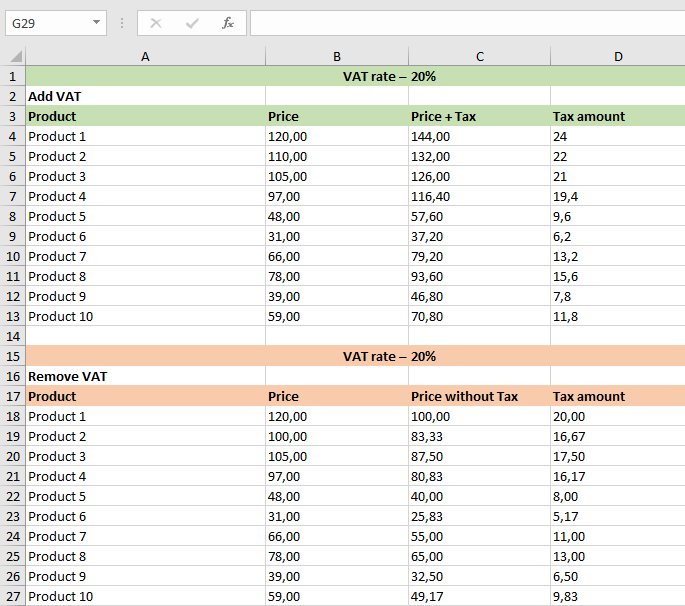# South African VAT Calculator

Updated on May 14, 2022

Our online VAT calculator in South Africa makes it easy to add or remove VAT. It’s as simple as typing in a price and pressing the button.

## What is VAT and how much is it in South Africa?

VAT (also known as GST in some countries) is a tax levied by EU member states on the sale of goods and services within their borders. VAT is now charged at a 15% standard rate. There are no reduced VAT rates.

sars.gov.za provides information on how to register for, calculate, pay, and reclaim VAT, VAT rates, and VAT on property rules for firms that are VAT-registered.

## How to work out VAT

Only 3 easy steps to calculate VAT onlinePlease double-check the “VAT rate” (which is now set at 15%)Specify whether the pricing is net or gross. The complete list of VAT rates can be found in the table below.## How to calculate VAT in math or Excel

You can use these simple procedures to calculate VAT in either direction.To account for VAT, simply multiply the price by 100 and divide the result by (100 + VAT rate). Including VAT – Gross price, that’s everything you need to know about the pricing.### Removing VAT formula

Divide the price by (100+VAT rate) and then multiply 100. Now that you’ve learned the price excluding VAT – the Net price, you can make an informed decision.

price.### VAT calculation in Excel

If you need to calculate VAT for several products at once, it is easier to use Excel. I created a template – download it, it’s free.

Categories VAT